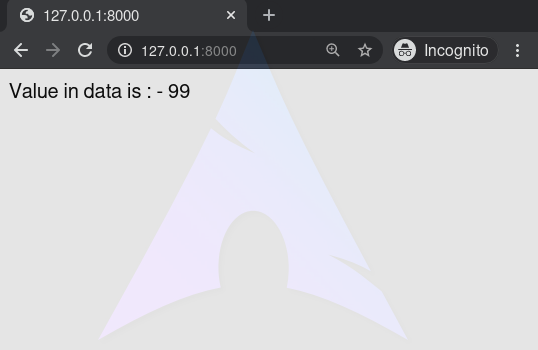# Boolean Operators – Django Template Tags

A Django template is a text document or a Python string marked-up using the Django template language. Django being a powerful Batteries included framework provides convenience to rendering data in a template. Django templates not only allow paassing data from view to template, but also provides some limited features of a programming such as variables, for loops, comments, extends, if else etc.
This article revolves about how to use boolean operators in Templates. The `{% if %}` tag evaluates a variable, and if that variable is “true” (i.e. exists, is not empty, and is not a false boolean value) the contents of the block are output. One can use various boolean operators with Django If Template tag.

###### Syntax
```{% if variable boolean_operator value %}
// statements
{% endif %}
```
###### Example

if tags may use and, or or not to test a number of variables or to negate a given variable:

 `{% if athlete_list and coach_list %} ` `    ``Both athletes and coaches are available. ` `{% endif %} ` ` `  `{% if not athlete_list %} ` `    ``There are no athletes. ` `{% endif %} ` ` `  `{% if athlete_list or coach_list %} ` `    ``There are some athletes or some coaches. ` `{% endif %} ` ` `  `{% if not athlete_list or coach_list %} ` `    ``There are no athletes or there are some coaches. ` `{% endif %} ` ` `  `{% if athlete_list and not coach_list %} ` `    ``There are some athletes and absolutely no coaches. ` `{% endif %} `

As one can see, the if tag may take one or several `{% elif %}` clauses, as well as an `{% else %}` clause that will be displayed if all previous conditions fail. These clauses are optional.

## Boolean operators – Django template Tags Explanation

Illustration of How to use Boolean operators in Django templates using an Example. Consider a project named `geeksforgeeks` having an app named `geeks`.

Refer to the following articles to check how to create a project and an app in Django.

Now create a view through which we will pass the context dictionary,
In `geeks/views.py`,

 `# import Http Response from django ` `from` `django.shortcuts ``import` `render ` `  `  `# create a function ` `def` `geeks_view(request): ` `    ``# create a dictionary ` `    ``context ``=` `{ ` `        ``"data"` `: ``99``, ` `    ``} ` `    ``# return response ` `    ``return` `render(request, ``"geeks.html"``, context) `

Create a url path to map to this view. In `geeks/urls.py`,

 `from` `django.urls ``import` `path ` ` `  `# importing views from views.py ` `from` `.views ``import` `geeks_view ` ` `  `urlpatterns ``=` `[ ` `    ``path('', geeks_view), ` `] `

Create a template in `templates/geeks.html`,

 `{% if data == 99 %} ` `Value in data is : - {{ data }} ` `{% else %} ` `Data is empty ` `{% endif%} `

Let’s check what is displayed on “/” are displayed in the template.## Boolean Operators

== operator
Equality. Example:

```{% if somevar == "x" %}
This appears if variable somevar equals the string "x"
{% endif %}
```

!= operator
Inequality. Example:

```{% if somevar != "x" %}
This appears if variable somevar does not equal the string "x",
{% endif %}```

< operator
Less than. Example:

```{% if somevar < 100 %}
This appears if variable somevar is less than 100.
{% endif %}```

> operator
Greater than. Example:

```{% if somevar > 0 %}
This appears if variable somevar is greater than 0.
{% endif %}```

<= operator
Less than or equal to. Example:

```{% if somevar <= 100 %}
This appears if variable somevar is less than 100 or equal to 100.
{% endif %}```

>= operator
Greater than or equal to. Example:

```{% if somevar >= 1 %}
This appears if variable somevar is greater than 1 or equal to 1.
{% endif %}```

in operator
Contained within. This operator is supported by many Python containers to test whether the given value is in the container. The following are some examples of how x in y will be interpreted:

```{% if "bc" in "abcdef" %}
This appears since "bc" is a substring of "abcdef"
{% endif %}```
```{% if "hello" in greetings %}
If greetings is a list or set, one element of which is the string
"hello", this will appear.
{% endif %}```
```{% if user in users %}
If users is a QuerySet, this will appear if user is an
instance that belongs to the QuerySet.
{% endif %}```

not in operator
Not contained within. This is the negation of the in operator.

is operator
Object identity. Tests if two values are the same object. Example:

```{% if somevar is True %}
This appears if and only if somevar is True.
{% endif %}

{% if somevar is None %}
This appears if somevar is None, or if somevar is not found in the context.
{% endif %}```

is not operator
Negated object identity. Tests if two values are not the same object. This is the negation of the is operator. Example:

```{% if somevar is not True %}
This appears if somevar is not True, or if somevar is not found in the
context.
{% endif %}

{% if somevar is not None %}
This appears if and only if somevar is not None.
{% endif %}```

Attention geek! Strengthen your foundations with the Python Programming Foundation Course and learn the basics.

To begin with, your interview preparations Enhance your Data Structures concepts with the Python DS Course.

My Personal Notes arrow_drop_upSoftware Developer at GeeksForGeeks

If you like GeeksforGeeks and would like to contribute, you can also write an article using contribute.geeksforgeeks.org or mail your article to contribute@geeksforgeeks.org. See your article appearing on the GeeksforGeeks main page and help other Geeks.

Please Improve this article if you find anything incorrect by clicking on the "Improve Article" button below.

Article Tags :

1

Please write to us at contribute@geeksforgeeks.org to report any issue with the above content.